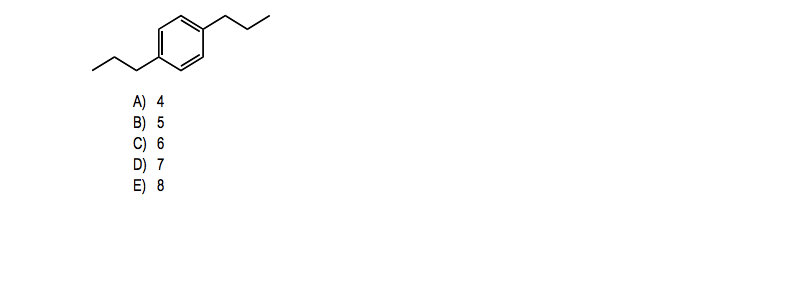# Problem: For the following compound how many different signals would you see in the proton NMR? (Assume that you can see them all)

###### FREE Expert Solution
79% (284 ratings)
###### Problem Details

For the following compound how many different signals would you see in the proton NMR? (Assume that you can see them all)Frequently Asked Questions

What scientific concept do you need to know in order to solve this problem?

Our tutors have indicated that to solve this problem you will need to apply the 1H NMR: Number of Signals concept. You can view video lessons to learn 1H NMR: Number of Signals. Or if you need more 1H NMR: Number of Signals practice, you can also practice 1H NMR: Number of Signals practice problems.

How long does this problem take to solve?

Our expert Organic tutor, Johnny took 4 minutes and 23 seconds to solve this problem. You can follow their steps in the video explanation above.

What professor is this problem relevant for?

Based on our data, we think this problem is relevant for Professor West's class at FAU.